# Hours Calculator

This calculator will take seconds to sum up work hours and minutes for you. You simply need to input the number of hours in the HH column and the number of minutes in the MM column. Using the "plus" sign, you can add another column of hours and minutes or remove it using the "minus" sign. The result sheet can be printed or emailed to you as well.

1. Use HH Text Box to Enter Hours.
2. Use MM Text Box to Enter Minutes.
3. Use Plus Sign Button to Another time duration.
4. Use Negative Sign Button to remove a Time Duration.
5. Results will be updated accordingly as you continue to add time.
6. Use Clear Button to clear All the Time entered.
7. Use Print Button To Print the complete Sheet.
8. Use Email Box if you want to recieve the complete hour sheet at your Email ID.

:
Total Time:

{{totalTime.totalHours + ':' + totalTime.totalMinutes}}About the Author Nauman Rehmat Nauman is a Digital Marketing Specialist and owner of several online tools like DrEmployee. He believes in helping common people by providing a free online solution to day to day tasks. This project is one of them to offer free financial tools and tips.

## How to Use Hours Calculator?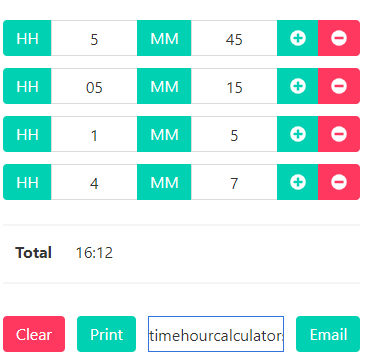Manually adding hours can be a tricky job; a single human error can cause a significant loss, for which you can use our Hour Calculator to get your work done in the most straightforward, easiest, and fastest way with accuracy.

Thanks to our user-friendly interface, you need to follow the steps to use our Hours Calculator:

Step 1:Upon launching Hours Calculator, you can see a single table row (As shown in the image above) where you have to enter Hours in textbox beside HH label and minutes in textbox beside MM label. This entry is your 1st value of the time duration.

Step 2:• Click on the Plus Sign button to add another row to enter another time duration. Repeat this step to add multiple time durations. Note: You can add up to 10 time-durations values in one go.
• Click on the Minus Sign button to remove/delete a time duration value.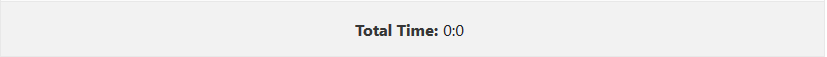• As shown in the image above, A sum (result) of each value you have entered is calculated instantly, and the result will appear beside Totallabel in HH:MM format where HH stands for Totals hours and MM stands for total minutes.

Step 3:

• You can click on the Clear button to reset the Calculator.
•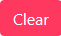• You can use Print button to print the entire Sheet.
•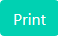• You can also email the complete Sheet by entering the recipient's email address and clicking on the Email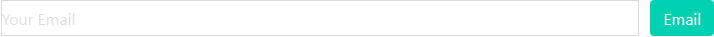## FAQs

There are numerous methods to calculate work hours. We are going to tell you some of the most effective and accurate methods with the least chances of human error.

To calculate Work Hours, you need to:

1. Record your daily Time IN and Time OUT of work by:
• Writing it manually on paper.
• OR stamping it on Timecard using a conventional time clock.
• OR recording it in Ms. Excel or any other spreadsheet software.
1. To manually calculate total work hours, you need to make sure, all values of Time In and Time Out are in Military Time format (24 Hours Format) for which can use our Military Time Converter.
2. After the conversion of time on Military Time format (24-Hours format), Subtract each Time In from the Time Out to calculate the Time Duration.
1. Now you will have a list of all time-durations you have worked in a Day or a Week or your desired number of days. Simple enter all durations in Hours Calculator located at the beginning of this page and get your Total Work Hours calculated instantaneously.

Note: If you don't want to get into the hassles of converting time into the 24-hour format and to manually calculate total work hours, then we recommend you to use our Time Card Calculatorto get your daily or weekly work hours calculated instantly using AM/PM time format.

To calculate your total number of hours worked and Pay, you need to follow the steps below:

1. First, you need to determine the time for which you need to calculate your pay, whether it is daily, weekly, monthly, or any of your desired period.
2. Record each Time In and Time Out of work within your determined period on a piece of paper, Ms. Excel, or other spreadsheets.
3. Convert your recorded Time in and Time out of work in a 24-Hours format using Military Time Converter.
4. Subtract each Time In from its Time Out to get time Duration of work using Time Elapsed Calculator. Now add up all your Time Durations of Work using Hours Calculator to get a total number of BASIC hours worked in your determined time.
5. Add Overtime & Double Time (If Any) to get a total payable number of hours worked
6. Now Multiply Total Payable Number of Hours Worked with your Hourly Rate to get your Gross Pay.
7. Now Add your Bonus / Commission / Tip Amount in your Gross Pay and subtract Withholding Tax and other charges from it (If any according to your employer’s and state’s Policy) to deduce your Net Pay for Total Number of Hours Worked in Determined Time.

Don't you have time?

The method mentioned above is the simple but lengthy method to calculate your Hours Worked and Pay. You can use our Hourly salary calculator to estimate your Gross Pay as well as Pay Check Calculator to calculate your Net Pay after Tax Deduction in a simple, precise, fast, and accurate way.

1. Sum up all the hours together.
2. Sum up all the minutes together.
3. If the summed-up value of minutes exceeds 60, then subtract 60 minutes from the minutes' value and add 1 hour in total hours. Repeat this step if the result value of minutes still exceeds 60.

To subtract Hours and Minutes, you can subtract them like typical values, but if the first minutes are less the one being subtracted, then follow these steps:

1. Add 1 Hour into the Original Hour.
2. Add 60 Minutes into Original Minutes.
3. Now subtract the minutes.
4. Now Subtract the hours.

1. Make a Table on a Paper, Ms. Excel, or any other spreadsheet to record your Time In and Time Out of work for a Week. You can also use the information on Time Card from a time clock if you have it.
• Ensure you are not counting the time of lunch breaks (if they are unpaid). Therefore, you will now have more than 1 Time in & Time Out times in a single day.
1. Make sure all your recorded times are in 24 Hours Format. If not, use our Military Time Convertor to get your work done instantly.
2. Subtract each Time In from Time Out to get total worked durations.
• Subtracting Time is not as simple as subtracting numerical values for which you are suggested to use our Time Elapsed Calculator.
1. Now you will have a list of Worked Durations. Add them manually by following the procedure stated in FAQs Question # 3 above OR use our Hours Calculator located at the beginning of this page, for instant and accurate total hours worked in a week.

Don't have time to follow so many steps? Don't Worry! We have the right tool for you. Use our Time Card Calculator to skip almost all of the steps above.

If you want to calculate your Hourly Rate, you need to determine your pay frequency; it is how often you are paid, whether it's weekly, bi-weekly, monthly, quarterly, or annually.

In the steps below, we are going to tell you the procedure to calculate the hourly rate from your weekly pay. You can use the same method to calculate through other pay frequencies as these calculations are based on Total Hours Worked. Here are the steps you need to follow:

1. To calculate your hourly rate, you need to figure out how many hours you have worked in a week. If you don’t know your total worked hours in a week, I suggest you to read an answer to FAQs Question # 4 above.
2. After figuring out your total hours worked in a week, you need to divide your weekly salary from total hours worked in a week.
• Basic Formula is Total Salary / Paid Hours, which you can use for any Pay Frequency.
Example:
• your total hours worked in a week are 48 hours and 50 minutes with your weekly salary of \$100. Then your hourly rate is 100/48.83 OR \$2.05 per hour.

#### Are you wondering why we have written 48 Hours and 50 Minutes as 48.83 in the worked example above?

It is because to divide salary from time, all values of time must be in the same form for which you need to convert minutes into decimal hours, and doing so is not that difficult. You need to divide your minutes from 60 to get decimal hours. So for the example above, we divided 50 by 60 to get 0.83 Hours.

You can also refer to the table below for quick Minutes to Decimal Hours Conversion: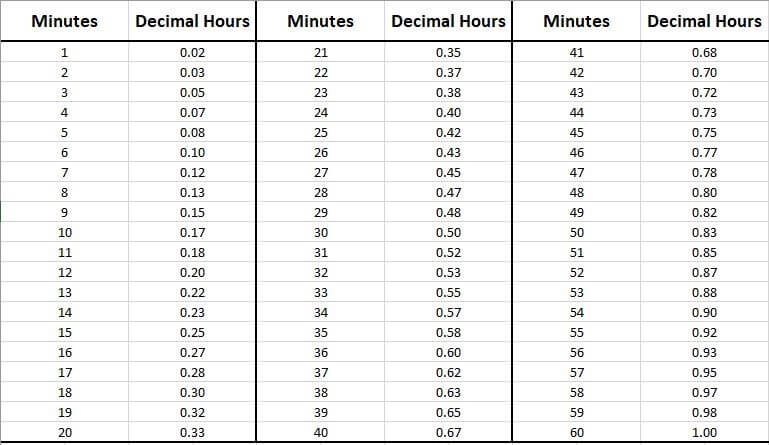Or you can also use our Hours to Decimal Converter for instant and error-free results.

There are numerous methods to calculate worked Hours per Month from which we have mentioned the most accurate one below:

#### Method 1: Average Hours Method

The first method can be used to get AVERAGE hours worked per month by following these steps:

1. Keep a record of Time In and Time Out of your work for ONE week, and follow the method stated in FAQs Question # 5, or you can also use our Time Card Calculator to calculate your Worked Hours in a Week.
2. Once you have figured out your Worked Hours in a Week, simply divide it by 7 to get your Average Daily Worked Hours.
3. Now multiply Average Daily Worked Hours by Number of Days in Month to get Average Hours Worked per Month.

Example:

If your Worked Hours in a Week are: 40.5 hours then:

Average Daily Worked Hours: 40.5 / 7 = 5.7 Hours

Hours Worked per Month: 5.7*30 = 173.5 Hours

#### Method 2: Record and Calculate Exact Hours

If you want the exact value of Hours worked in a Month then you are suggested to follow the steps below:

1. Make a Spreadsheet and Keep a record of your Time Inand Time Out of Work.

Note: If your Lunch Breaks are unpaid, then you shouldn’t include that time in your spreadsheet. Therefore, you will have More than one Time In and Time Outs in a Day.

1. Now calculate your Total Hours Worked in a Week by using any ONE method below:
1. Our Two Shift Calculator if you have Unpaid Lunch Breaks.
2. OROur Time Card Calculator if you have Paid Lunch Breaks.
3. ORYou can also use our Bi-Weekly timesheet Calculator if you want to calculate Total Hours Worked in Two Weeks.
4. ORFollow the Method stated in FAQs Question # 5 above.
1. Repeat Step # 2, for every week in a month to get four values of Total Hours Worked in a Week.
2. As there are more days in a month than days in 4 weeks (28 Days), so you can still use calculators mentioned in Step # 2 to get Hours Worked in Remaining Days.
3. Now Add All 4 Values of Total Hours Worked in a Week and a Value of Hours Worked in Remaining Days together using our Hour Calculator located at the beginning of the page to get Exact Value of Hours worked in a Month.

#### Method 1: Don’t record lunch break time duration

To calculate hours worked minus lunch, you should not record Work Time during Lunch. Therefore, you will have more than One Time IN and Time OUT of work in a day.

Now use our Two Shift Calculator to calculate all your Total Hours Worked minus Lunch in a Week.

#### Method 2: Subtract your Fixed Lunch Break Time

If you have total hours worked, including lunch break, and you have a fixed Lunch Break time like 30 Minutes daily than you can subtract total your Total lunch break time from total hours worked.

Example:

Hours Worked in a Week (including Lunch Breaks): 40.5 Hours

Daily Lunch Break Time: 30 Minutes

Lunch Break Time of a Week (In Minutes): 30*7 = 210 Minutes.

Lunch Break Time of a Week (In Minutes): 210/60= 3.5 Hours

Total Hours Worked minus Lunch in a Week: 40.5-3.5= "37 Hours".

Other Calcutors